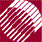ECE532 Biomedical Optics © 1998 Steven L. Jacques, Scott A. Prahl Oregon Graduate Institute

Photon drops weight due to absorption

The photon now interacts with the tissue. The first interaction is to drop some photon weight due to absorption and assign that weight to the local bin which accumulates dropped photon weight from all the launched photons.

The albedo equals the fractional probablility of being scattered and 1 - albedo equals the fractional probablility of being absorbed. So a fraction of the current photon weight is absorbed, absorb = W*(1 - albedo), and the current photon weight is reduced by this same amount, W = W - absorb. The remaining weight will be scattered in the next section called SPIN.

The absorbed weight is dropped into three different bins associated with the spherical, cylindrical, and planar arrays Csph[ir], Ccyl[ir], Cpla[ir] where ir is an index integer which denotes the distance (r) from the source to the bin. The value of r is different for the three dimensions of accumulation.

If the index value ir exceeds the maximum bin number NR, then the absorbed photon weight is deposited in the last bin which serves as an overflow bin. Summing all the bins including the overflow bin allows one to check that conservation of photon energy holds.

/**** DROP
Drop photon weight (W) into local bin.
*****/
absorb = W*(1 - albedo);      /* photon weight absorbed at this step */
W -= absorb;                  /* decrement WEIGHT by amount absorbed */

/* spherical */
r = sqrt(x*x + y*y + z*z);    /* current spherical radial position */
ir = (short)(r/dr);           /* ir = index to spatial bin */
if (ir >= NR) ir = NR;        /* last bin is for overflow */
Csph[ir] += absorb;           /* DROP absorbed weight into bin */

/* cylindrical */
r = sqrt(x*x + y*y);          /* current cylindrical radial position */
ir = (short)(r/dr);           /* ir = index to spatial bin */
if (ir >= NR) ir = NR;        /* last bin is for overflow */
Ccyl[ir] += absorb;           /* DROP absorbed weight into bin */

/* planar */
r = fabs(z);                  /* current planar radial position */
ir = (short)(r/dr);           /* ir = index to spatial bin */
if (ir >= NR) ir = NR;        /* last bin is for overflow */
Cpla[ir] += absorb;           /* DROP absorbed weight into bin */

to next page | Chapter 4 | Course | Home

©1998, Steven L. Jacques, Scott A. Prahl, Oregon Graduate Institute, Oregon Medical Laser Center